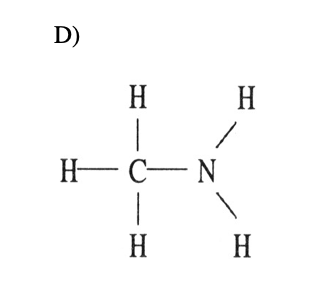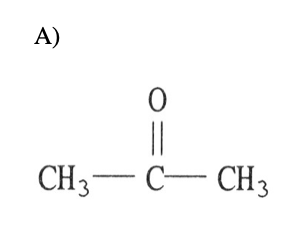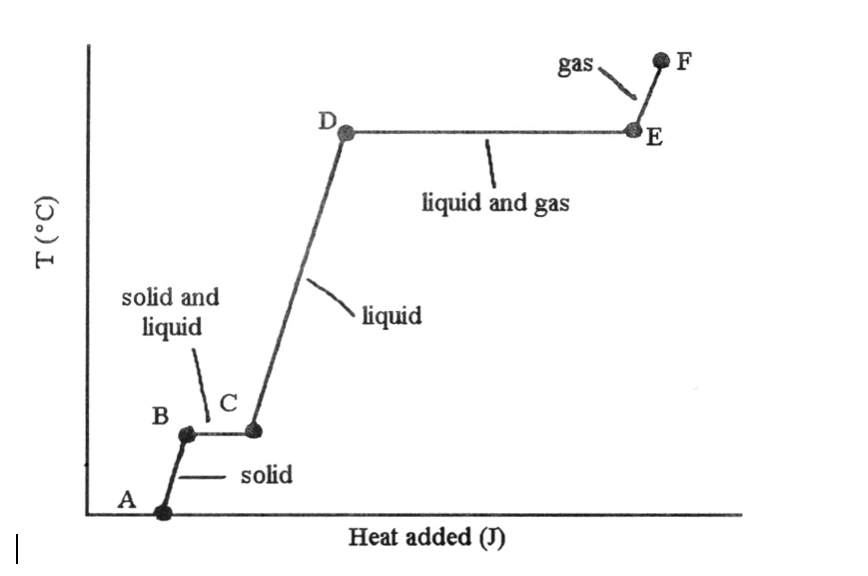# Chemistry: Ch 11: Chem Study Packet Flashcards

Set Details Share
created 5 years ago by jessjess2601
24,381 views
updated 5 years ago by jessjess2601
Subjects:
science, chemistry
Page to share:
Embed this setcancel
COPY
code changes based on your size selection
Size:
X

1

1) Crystalline solids ________.

A) have their particles arranged randomly

B) have highly ordered structures

C) are usually very soft

D) exist only at high temperatures

E) exist only at very low temperatures

B) have highly ordered structures

2

2) In liquids, the attractive intermolecular forces are ________.

A) very weak compared with kinetic energies of the molecules

B) strong enough to hold molecules relatively close together

C) strong enough to keep the molecules confined to vibrating about their fixed lattice points

D) not strong enough to keep molecules from moving past each other

E) strong enough to hold molecules relatively close together but not strong enough to keep molecules from moving past each other

E) strong enough to hold molecules relatively close together but not strong enough to keep molecules from moving past each other

3

3) As a gaseous element condenses, the atoms become ________ and they have ________ attraction for one another.

A) more separated, more

B) more separated, less

C) closer together, more

D) closer together, less

E) larger, greater

C) closer together, more

4

4) A gas is ________ and assumes ________ of its container, whereas a liquid is ________ and assumes ________ of its container.

A) compressible, the volume and shape, not compressible, the shape of a portion

B) compressible, the shape, not compressible, the volume and shape

C) compressible, the volume and shape, compressible, the volume

D) condensed, the volume and shape, condensed, the volume and shape

E) condensed, the shape, compressible, the volume and shape

A) compressible, the volume and shape, not compressible, the shape of a portion

5

5) Together, liquids and solids constitute ________ phases of matter.

A) the compressible

B) the fluid

C) the condensed

D) all of the

E) the disordered

C) the condensed

6

6) Which statement is true about liquids but not true about solids?

A) They flow and are highly ordered.

B) They are highly ordered and not compressible.

C) They flow and are compressible.

D) They assume both the volume and the shape of their containers.

E) They flow and are not compressible.

C) They flow and are compressible.

7

7) The strongest interparticle attractions exist between particles of a ________, and the weakest interparticle attractions exist between particles of a ________.

A) solid, liquid

B) solid, gas

C) liquid, gas

D) liquid, solid

E) gas, solid

B) solid, gas

8

8) Of the following substances, only ________ has London dispersion forces as the only intermolecular force.

A) CH3OH

B) NH3

C) H2S

D) Kr

E) HCl

D) Kr

9

9) In which of the following molecules is hydrogen bonding likely to be the most significant component of the total intermolecular forces?

A) CH4

B) C5H11OH

C) C6H13NH2

D) CH3OH

E) CO2

D) CH3OH

10

10) Which of the following has dispersion forces as its only intermolecular force?

A) CH4

B) HCl

C) C6H13NH2

D) NaCl

E) CH3Cl

A) CH4

11

11) When NaCl dissolves in water, aqueous Na+ and Cl- ions result. The force of attraction that exists between Na+ and H2O is called a(n) ________ interaction.

A) dipole-dipole

B) ion-ion

C) hydrogen bonding

D) ion-dipole

E) London dispersion force

D) ion-dipole

12

12) ________ are particularly polarizable.

A) Small nonpolar molecules

B) Small polar molecules

C) Large nonpolar molecules

D) Large polar molecules

E) Large molecules, regardless of their polarity,

E) Large molecules, regardless of their polarity,

13

13) The ease with which the charge distribution in a molecule can be distorted by an external electrical field is called the ________.

A) electronegativity

B) hydrogen bonding

C) polarizability

D) volatility

E) viscosity

C) polarizability

14

14) The intermolecular force(s) responsible for the fact that CH4 has the lowest boiling point in the set CH4, SiH4, GeH4, SnH4 is/are ________.

A) hydrogen bonding

B) dipole-dipole interactions

C) London dispersion forces

D) mainly hydrogen bonding but also dipole-dipole interactions

E) mainly London-dispersion forces but also dipole-dipole interactions

C) London dispersion forces

15

15) Elemental iodine (I2) is a solid at room temperature. What is the major attractive force that exists among different I2 molecules in the solid?

A) London dispersion forces

B) dipole-dipole rejections

C) ionic-dipole interactions

D) covalent-ionic interactions

E) dipole-dipole attractions

A) London dispersion forces

16

16) Hydrogen bonding is a special case of ________.

A) London-dispersion forces

B) ion-dipole attraction

C) dipole-dipole attractions

D) ion-ion interactions

E) none of the above

C) dipole-dipole attractions

17

17) Which one of the following substances will have hydrogen bonding as one of its intermolecular forces?18

18) Which one of the following substances will not have hydrogen bonding as one of its intermolecular forces?19

19) What intermolecular force is responsible for the fact that ice is less dense than liquid water?

A) London dispersion forces

B) dipole-dipole forces

C) ion-dipole forces

D) hydrogen bonding

E) ionic bonding

D) hydrogen bonding

20

20) The predominant intermolecular force in (CH3)2NH is ________.

A) London dispersion forces

B) ion-dipole forces

C) ionic bonding

D) dipole-dipole forces

E) hydrogen bonding

E) hydrogen bonding

21

21) C12H26 molecules are held together by ________.

A) ion-ion interactions

B) hydrogen bonding

C) ion-dipole interactions

D) dipole-dipole interactions

E) dispersion forces

E) dispersion forces

22

22) Which of the following molecules has hydrogen bonding as its only intermolecular force?

A) HF

B) H2O

C) C6H13NH2

D) C5H11OH

E) None, all of the above exhibit dispersion forces.

E) None, all of the above exhibit dispersion forces.

23

23) Which of the following molecules has hydrogen bonding as its only intermolecular force?

A) NH3

B) H2O

C) C3H7OH

D) HOCH2CH2OH

E) None, all of the above exhibit dispersion forces.

E) None, all of the above exhibit dispersion forces.

24

24) What types of intermolecular forces exist between HI and H2S?

A) dipole-dipole and ion-dipole

B) dispersion forces, dipole-dipole, and ion-dipole

C) dispersion forces, hydrogen bonding, dipole-dipole, and ion-dipole

D) dispersion forces and dipole-dipole

E) dispersion forces and ion-dipole

D) dispersion forces and dipole-dipole

25

5) What type(s) of intermolecular forces exist between Br2 and CCl4?

A) dispersion forces

B) dispersion forces and ion-dipole

C) dispersion forces and dipole-dipole

D) dispersion forces, ion-dipole, and dipole-dipole

E) None. Since both are gases at room temperature, they do not interact with each other.

A) dispersion forces

26

26) What type(s) of intermolecular forces exist between PH3 and CO3 2 -?

A) dispersion forces

B) dispersion forces and ion-dipole

C) dispersion forces, ion-dipole, and dipole-dipole

D) dispersion forces and dipole-dipole

E) dispersion forces, ion-dipole, dipole-dipole, and hydrogen bonds

B) dispersion forces and ion-dipole

27

27) What types of intermolecular forces exist between NH3 and H2S?

A) dispersion forces and dipole-dipole forces

B) dispersion forces

C) dispersion forces and hydrogen bonds

D) dispersion forces, hydrogen bonds, and dipole-dipole forces

E) dispersion forces, hydrogen bonds, and ion-dipole forces

A) dispersion forces and dipole-dipole forces

28

28) What types of intermolecular forces exist between NH3 and HF?

A) dispersion forces and dipole-dipole forces

B) dispersion forces and hydrogen bonds

C) dispersion forces, dipole-dipole forces, and hydrogen bonds

D) dispersion forces

E) dispersion forces, hydrogen bonds, and ion-dipole forces

C) dispersion forces, dipole-dipole forces, and hydrogen bonds

29

29) ________ is the energy required to expand the surface area of a liquid by a unit amount of area.

A) Viscosity

B) Surface tension

C) Volatility

D) Meniscus

E) Capillary action

B) Surface tension

30

30) Which statements about viscosity are true?

(i) Viscosity increases as temperature decreases.

(ii) Viscosity increases as molecular weight increases.

(iii) Viscosity increases as intermolecular forces increase.

A) (i) only

B) (ii) and (iii)

C) (i) and (iii)

D) none

E) all

E) all

31

31) The shape of a liquid's meniscus is determined by ________.

A) the viscosity of the liquid

B) the type of material the container is made of

C) the relative magnitudes of cohesive forces in the liquid and adhesive forces between the liquid and its container

D) the amount of hydrogen bonding in the liquid

E) the volume of the liquid

C) the relative magnitudes of cohesive forces in the liquid and adhesive forces between the liquid and its container

32

32) Viscosity is ________.

A) the "skin" on a liquid surface caused by intermolecular attraction

B) the resistance to flow

C) the same as density

D) inversely proportional to molar mass

E) unaffected by temperature

B) the resistance to flow

33

33) How high a liquid will rise up a narrow tube as a result of capillary action depends on ________.

A) the magnitudes of cohesive forces in the liquid and adhesive forces between the liquid and the tube, and gravity

B) gravity alone

C) only the magnitude of adhesive forces between the liquid and the tube

D) the viscosity of the liquid

E) only the magnitude of cohesive forces in the liquid

A) the magnitudes of cohesive forces in the liquid and adhesive forces between the liquid and the tube, and gravity

34

34) The property responsible for the "beading up" of water is ________.

A) density

B) viscosity

C) vapor pressure

D) surface tension

E) hydrogen bonding

D) surface tension

35

35) Heat of sublimation can be approximated by adding together ________ and ________.

A) heat of fusion, heat of condensation

B) heat of fusion, heat of vaporization

C) heat of freezing (solidification), heat of condensation

D) heat of freezing (solidification), heat of vaporization

E) heat of deposition, heat of vaporization

B) heat of fusion, heat of vaporization

36

36) Which of the following statements is false?

A) The absolute value of the heat of sublimation is equal to the absolute value of the heat of deposition.

B) The heat of sublimation is equal to the sum of the heat of vaporization and the heat of melting.

C) The heat of sublimation is equal to the sum of the heat of vaporization and the heat of freezing.

D) The absolute value of the heat of sublimation is equal to the absolute value of the sum of the heat of condensation and the heat of freezing.

E) The absolute value of the heat of deposition is equal to sum of the absolute value of the heat of vaporization and the absolute value of the heat of freezing.

C) The heat of sublimation is equal to the sum of the heat of vaporization and the heat of freezing.

3737) The phase changes B → C and D → E are not associated with temperature increases because the heat energy is used up to ________.

A) increase distances between molecules

B) break intramolecular bonds

C) rearrange atoms within molecules

D) increase the velocity of molecules

E) increase the density of the sample

A) increase distances between molecules

38

38) Based on the following information, which compound has the strongest intermolecular forces?

Substance ΔH vap (kJ/mol)

Argon (Ar) 6.3

Benzene (C6H6)31.0

Ethanol (C2H5OH)39.3

Water (H2O)40.8

Methane (CH4)9.2

A) Argon

B) Benzene

C) Ethanol

D) Water

E) Methane

D) Water

39

39) Which compound has the strongest intermolecular forces?

A) CBr4

B) C12H26

C) CI4

D) N2

E) O2

C) CI4

40

40) Large intermolecular forces in a substance are manifested by ________.

A) low vapor pressure

B) high boiling point

C) high heats of fusion and vaporization

D) high critical temperatures and pressures

E) all of the above

E) all of the above

41

41) A substance that expands to fill its container yet has a density approaching that of a liquid, and that can behave as a solvent is called a(n) ________.

A) plasma

B) gas

C) liquid

D) amorphous solid

E) supercritical fluid and gas

E) supercritical fluid and gas

42

42) The critical temperature and pressure of CS2 are 279 °C and 78 atm, respectively. At temperatures above 279 °C and pressures above 78 atm, CS2 can only occur as a ________.

A) solid

B) liquid

C) liquid and gas

D) gas

E) supercritical fluid

E) supercritical fluid

43

43) The substance with the largest heat of vaporization is ________.

A) I2

B) Br2

C) Cl2

D) F2

E) O2

A) I2

44

44) Of the following, ________ is an exothermic process.

A) melting

B) subliming

C) freezing

D) boiling

E) All of the above are exothermic.

C) freezing

45

45) Of the following, ________ should have the highest critical temperature.

A) CBr4

B) CCl4

C) CF4

D) CH4

E) H2

A) CBr4

46

46) A volatile liquid is one that ________.

A) is highly flammable

B) is highly viscous

C) is highly hydrogen-bonded

D) is highly cohesive

47

47) In general, the vapor pressure of a substance increases as ________ increases.

A) surface tension

B) molecular weight

C) hydrogen bonding

D) viscosity

E) temperature

E) temperature

48

48) The vapor pressure of any substance at its normal boiling point is

A) 1 Pa

B) 1 torr

C) 1 atm

D) equal to atmospheric pressure

E) equal to the vapor pressure of water

C) 1 atm

49

49) Volatility and vapor pressure are ________.

A) inversely proportional to one another

B) directly proportional to one another

C) not related

D) the same thing

E) both independent of temperature

B) directly proportional to one another

50

50) Some things take longer to cook at high altitudes than at low altitudes because ________.

A) water boils at a lower temperature at high altitude than at low altitude

B) water boils at a higher temperature at high altitude than at low altitude

C) heat isn't conducted as well in low density air

D) natural gas flames don't burn as hot at high altitudes

E) there is a higher moisture content in the air at high altitude

A) water boils at a lower temperature at high altitude than at low altitude

51

51) The vapor pressure of a liquid ________.

A) increases linearly with increasing temperature

B) increases nonlinearly with increasing temperature

C) decreases linearly with increasing temperature

D) decreases nonlinearly with increasing temperature

E) is totally unrelated to its molecular structure

B) increases nonlinearly with increasing temperature

52

53) On a phase diagram, the critical pressure is ________.

A) the pressure required to melt a solid

B) the pressure below which a substance is a solid at all temperatures

C) the pressure above which a substance is a liquid at all temperatures

D) the pressure at which a liquid changes to a gas

E) the pressure required to liquefy a gas at its critical temperature

E) the pressure required to liquefy a gas at its critical temperature

53

54) On a phase diagram, the critical temperature is ________.

A) the temperature below which a gas cannot be liquefied

B) the temperature above which a gas cannot be liquefied

C) the temperature at which all three states are in equilibrium

D) the temperature required to melt a solid

E) the temperature required to cause sublimation of a solid

B) the temperature above which a gas cannot be liquefied

54

55) On a phase diagram, the melting point is the same as ________.

A) the triple point

B) the critical point

C) the freezing point

D) the boiling point

E) the vapor-pressure curve

C) the freezing point

55

56) When the phase diagram for a substance has a solid-liquid phase boundary line that has a negative slope (leans to the left), the substance ________.

A) can go from solid to liquid, within a small temperature range, via the application of pressure

B) sublimes rather than melts under ordinary conditions

C) cannot go from solid to liquid by application of pressure at any temperature

D) cannot be liquefied above its triple point

E) melts rather than sublimes under ordinary conditions

A) can go from solid to liquid, within a small temperature range, via the application of pressure

56

57) The predominant intermolecular force in CaBr2 is ________.

A) London-dispersion forces

B) ion-dipole forces

C) ionic bonding

D) dipole-dipole forces

E) hydrogen bonding

C) ionic bonding

57

58) Which of the following is most likely to exhibit liquid-crystalline behavior?

A) CH3CH2-C(CH3)2-CH2CH3

B) CH3CH2CH2CH2CH2CH2CH2CH3

C) CH3CH2CH2CH2CH2-

D)

E)

e

58

59) Which of the following characteristics would prevent liquid crystal behavior?

A) long axial structure

B) ionic configuration

C) carbon-carbon single bonds

D) double bonding

E) polar groups

B) ionic configuration

59

60) In the smectic A liquid-crystalline phase, ________.

A) the molecules are aligned along their long axes, with no ordering with respect to the ends of the molecules

B) the molecules are arranged in sheets, with their long axes parallel and their ends aligned as well

C) the molecules are aligned with their long axes tilted with respect to a line perpendicular to the plane in which the molecules are stacked

D) disk-shaped molecules are aligned through a stacking of the disks in layers

E) the molecules are oriented in a totally random fashion

B) the molecules are arranged in sheets, with their long axes parallel and their ends aligned as well